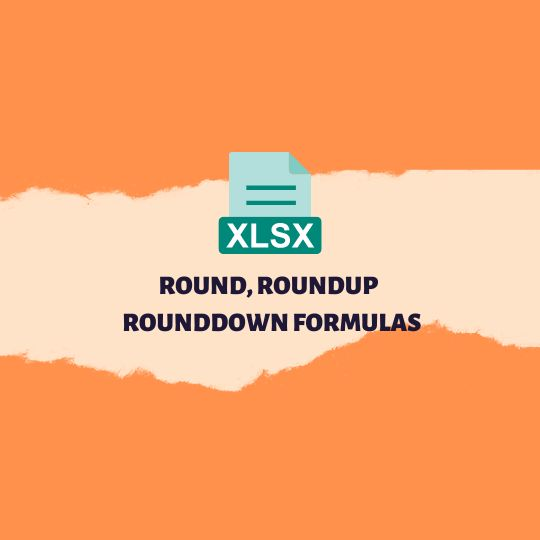# Round, Roundup and Rounddown FormulaskeySkillset
30
November
2022### What is Round, Roundup, and Rounddown?

In this blog, we will look at the formula, Round, Roundup, and Rounddown that are variations. If you are working a lot with the numbers in Excel, you probably came across with the problem where you wanted to round the numbers to the closest number. You probably even tried to reduce the decimal places to make it look like rounded. However, if you want your numbers to be rounded to the closest number it is, this is where Round formulas come in.

### Round

Round is a simple formula which works with two different variables. In the examples below, you can see that Round formula uses two different inputs, the number, and the decimal. The number variable that we selected for Round formula is the one that we want to round. The decimal place variable asks us how many decimals we want to keep after the rounding operation.

### Roundup and Rounddown

The two variations of round function are similar to the Round formula. For both Roundup and Rounddown formulas, we have the same to variables, the number, and the decimal point. The difference between round and those two formulas is roundup and Rounddown round the number to the upper or the lower limit. If you use Roundup formula, instead of rounding the number to the closest number, it will round the decimal up. If you use Rounddown formula, the formula will round the number down.

For example, if you use round formula for 3.4 and 3.7, you will get two different numbers as an outcome. For 3.4 you will get 3 and for 3.7 you will get 4.

However, if you use Roundup for those number, the outcome will be 4 for both of them. And if you use Rounddown, it will give you the result 3 for both of these numbers.

The Round (Roundup and Rounddown) formula in Excel is a useful tool for easily rounding numbers to the nearest whole number, tenth, hundredth, or thousandth. It is a simple formula that can save time and make calculations easier.